# Resources tagged with: Fractions

Filter by: Content type:
Age range:
Challenge level:

### There are 64 results

Broad Topics > Fractions, Decimals, Percentages, Ratio and Proportion > Fractions##### Age 11 to 14Challenge Level

Aisha's division and subtraction calculations both gave the same answer! Can you find some more examples?### Couples

##### Age 11 to 14Challenge Level

In a certain community two thirds of the adult men are married to three quarters of the adult women. How many adults would there be in the smallest community of this type?### The Greedy Algorithm

##### Age 11 to 14Challenge Level

The Egyptians expressed all fractions as the sum of different unit fractions. The Greedy Algorithm might provide us with an efficient way of doing this.### Water Lilies

##### Age 11 to 14Challenge Level

There are some water lilies in a lake. The area that they cover doubles in size every day. After 17 days the whole lake is covered. How long did it take them to cover half the lake?### Counting Fish

##### Age 14 to 16Challenge Level

I need a figure for the fish population in a lake. How does it help to catch and mark 40 fish?### Tweedle Dum and Tweedle Dee

##### Age 11 to 14Challenge Level

Two brothers were left some money, amounting to an exact number of pounds, to divide between them. DEE undertook the division. "But your heap is larger than mine!" cried DUM...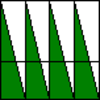### Teaching Fractions with Understanding: Part-whole Concept

##### Age 5 to 14

Written for teachers, this article describes four basic approaches children use in understanding fractions as equal parts of a whole.### Tiny Nines

##### Age 14 to 16Challenge Level

What do you notice about these families of recurring decimals?### Repetitiously

##### Age 14 to 16Challenge Level

Can you express every recurring decimal as a fraction?### Terminating or Not

##### Age 11 to 14Challenge Level

Is there a quick way to work out whether a fraction terminates or recurs when you write it as a decimal?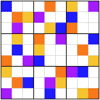### Ratio Sudoku 3

##### Age 11 to 16Challenge Level

A Sudoku with clues as ratios or fractions.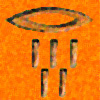### History of Fractions

##### Age 7 to 14

Who first used fractions? Were they always written in the same way? How did fractions reach us here? These are the sorts of questions which this article will answer for you.### More Twisting and Turning

##### Age 11 to 16Challenge Level

It would be nice to have a strategy for disentangling any tangled ropes...### Twisting and Turning

##### Age 11 to 14Challenge Level

Take a look at the video and try to find a sequence of moves that will untangle the ropes.### Rod Fractions

##### Age 7 to 14Challenge Level

Pick two rods of different colours. Given an unlimited supply of rods of each of the two colours, how can we work out what fraction the shorter rod is of the longer one?### Pythagoras’ Comma

##### Age 14 to 16Challenge Level

Using an understanding that 1:2 and 2:3 were good ratios, start with a length and keep reducing it to 2/3 of itself. Each time that took the length under 1/2 they doubled it to get back within range.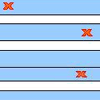### Stretching Fractions

##### Age 14 to 16Challenge Level

Imagine a strip with a mark somewhere along it. Fold it in the middle so that the bottom reaches back to the top. Stetch it out to match the original length. Now where's the mark?### Circuit Training

##### Age 14 to 16Challenge Level

Mike and Monisha meet at the race track, which is 400m round. Just to make a point, Mike runs anticlockwise whilst Monisha runs clockwise. Where will they meet on their way around and will they ever. . . .### Harmonic Triangle

##### Age 14 to 16Challenge Level

Can you see how to build a harmonic triangle? Can you work out the next two rows?### Matching Fractions, Decimals and Percentages

##### Age 7 to 14Challenge Level

Can you match pairs of fractions, decimals and percentages, and beat your previous scores?### Special Sums and Products

##### Age 11 to 14Challenge Level

Find some examples of pairs of numbers such that their sum is a factor of their product. eg. 4 + 12 = 16 and 4 × 12 = 48 and 16 is a factor of 48.### Sum Equals Product

##### Age 11 to 14Challenge Level

The sum of the numbers 4 and 1 [1/3] is the same as the product of 4 and 1 [1/3]; that is to say 4 + 1 [1/3] = 4 ï¿½ 1 [1/3]. What other numbers have the sum equal to the product and can this be. . . .### Keep it Simple

##### Age 11 to 14Challenge Level

Can all unit fractions be written as the sum of two unit fractions?### Countdown Fractions

##### Age 11 to 16Challenge Level

Here is a chance to play a fractions version of the classic Countdown Game.### Fractions and Percentages Card Game

##### Age 11 to 16Challenge Level

Can you find the pairs that represent the same amount of money?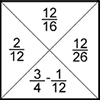### Fractions Jigsaw

##### Age 11 to 14Challenge Level

A jigsaw where pieces only go together if the fractions are equivalent.### Smaller and Smaller

##### Age 7 to 14Challenge Level

Can you predict, without drawing, what the perimeter of the next shape in this pattern will be if we continue drawing them in the same way?### Fair Shares?

##### Age 14 to 16Challenge Level

A mother wants to share a sum of money by giving each of her children in turn a lump sum plus a fraction of the remainder. How can she do this in order to share the money out equally?### How Long Is the Cantor Set?

##### Age 11 to 14Challenge Level

Take a line segment of length 1. Remove the middle third. Remove the middle thirds of what you have left. Repeat infinitely many times, and you have the Cantor Set. Can you find its length?### Farey Sequences

##### Age 11 to 14Challenge Level

There are lots of ideas to explore in these sequences of ordered fractions.### Rationals Between...

##### Age 14 to 16Challenge Level

What fractions can you find between the square roots of 65 and 67?### Egyptian Fractions

##### Age 11 to 14Challenge Level

The Egyptians expressed all fractions as the sum of different unit fractions. Here is a chance to explore how they could have written different fractions.### Hello Again

##### Age 11 to 14Challenge Level

Anne completes a circuit around a circular track in 40 seconds. Brenda runs in the opposite direction and meets Anne every 15 seconds. How long does it take Brenda to run around the track?### Unit Fractions

##### Age 11 to 14Challenge Level

Consider the equation 1/a + 1/b + 1/c = 1 where a, b and c are natural numbers and 0 < a < b < c. Prove that there is only one set of values which satisfy this equation.### Almost One

##### Age 11 to 14Challenge Level

Choose some fractions and add them together. Can you get close to 1?### Equal Temperament

##### Age 14 to 16Challenge Level

The scale on a piano does something clever : the ratio (interval) between any adjacent points on the scale is equal. If you play any note, twelve points higher will be exactly an octave on.### Lower Bound

##### Age 14 to 16Challenge Level

What would you get if you continued this sequence of fraction sums? 1/2 + 2/1 = 2/3 + 3/2 = 3/4 + 4/3 =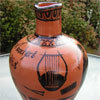### Six Notes All Nice Ratios

##### Age 14 to 16Challenge Level

The Pythagoreans noticed that nice simple ratios of string length made nice sounds together.### Fractions Rectangle

##### Age 11 to 14Challenge Level

The large rectangle is divided into a series of smaller quadrilaterals and triangles. Can you untangle what fractional part is represented by each of the ten numbered shapes?### Tangles

##### Age 11 to 16

A personal investigation of Conway's Rational Tangles. What were the interesting questions that needed to be asked, and where did they lead?### Archimedes and Numerical Roots

##### Age 14 to 16Challenge Level

The problem is how did Archimedes calculate the lengths of the sides of the polygons which needed him to be able to calculate square roots?### 3388

##### Age 11 to 14Challenge Level

Using some or all of the operations of addition, subtraction, multiplication and division and using the digits 3, 3, 8 and 8 each once and only once make an expression equal to 24.### Fracmax

##### Age 14 to 16Challenge Level

Find the maximum value of 1/p + 1/q + 1/r where this sum is less than 1 and p, q, and r are positive integers.### A Chance to Win?

##### Age 11 to 14Challenge Level

Imagine you were given the chance to win some money... and imagine you had nothing to lose...### Racing Odds

##### Age 11 to 14Challenge Level

In a race the odds are: 2 to 1 against the rhinoceros winning and 3 to 2 against the hippopotamus winning. What are the odds against the elephant winning if the race is fair?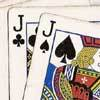### Do Unto Caesar

##### Age 11 to 14Challenge Level

At the beginning of the night three poker players; Alan, Bernie and Craig had money in the ratios 7 : 6 : 5. At the end of the night the ratio was 6 : 5 : 4. One of them won \$1 200. What were the. . . .### All Tangled Up

##### Age 14 to 18Challenge Level

Can you tangle yourself up and reach any fraction?### Diminishing Returns

##### Age 11 to 14Challenge Level

How much of the square is coloured blue? How will the pattern continue?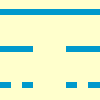### The Cantor Set

##### Age 11 to 14Challenge Level

Take a line segment of length 1. Remove the middle third. Remove the middle thirds of what you have left. Repeat infinitely many times, and you have the Cantor Set. Can you picture it?### Cuisenaire Environment

##### Age 5 to 16Challenge Level

An environment which simulates working with Cuisenaire rods.